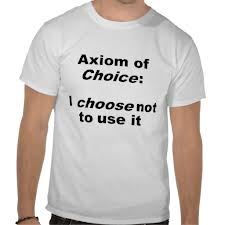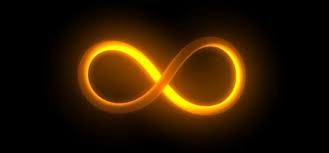# Axiomatic Set Theory

Sets were introduced towards the end of the nineteenth century and it was quickly realised that they could be a suitable foundation on which to build the whole of mathematics. The basic assumption was that for every property P we can form the set of all things having that property, that is {x | Px}, or so it was assumed. But the Russell paradox showed that this is not justified for if S = {x | x ∉ x} then S ∈ S if and only if S ∉ S.Axiomatic Set Theory was developed as a way of avoiding this pitfall, by legislating which properties can be allowed to give rise to sets. There were several ways proposed for doing this, all of which are equivalent to one another. In these notes we build upon the Zermelo Fraenkel Axioms.

We show how, assuming the ZF axioms, we can develop a set theory in which everything we need is a set. Numbers can be defined as sets, ordered pairs can be defined as sets, functions can be defined as sets. Indeed every object in mathematics can be defined as a set.

Essentially every mathematical theorem can be proved by building on the ZF axioms, though we don't attempt to do that! But we sketch how this can be done by developing the natural numbers, the positive rational numbers, the positive reals, the set of all real numbers and finally the complex field. All of these numbers are defined as sets of sets ...

Our task is to show how all of mathematics can be developed in the ZF context, and this means we must avoid using geometric intuition. Angles, and the trigonometric functions are tricky in this respect. But we define the trigonometric functions by their power series and define Π by Π/2 being the smallest positive x for which cos x = 0.Then we go beyond into the realms of the infinite where we define the cardinal number of a set and show that there are many different sizes of infinity. The Axiom of Choice is discussed, along with a number of equivalent statements. This is consistent with, but independent of the ZF axioms, which means we are logically free to accept or reject it. Its statement seems intuitively obvious yet it can be used to prove theorems that seem intuitively false!

Finally we discuss ordered sets, and in particular well-ordered sets, leading to the theory of infinite ordinal numbers.

A study of Axiomatic Set Theory causes one to look at the nature of mathematical truth and takes one to bizarre corners of the mathematical universe where logic survives, but only just!

[Please note that all links are to Adobe .pdf documents. They will open in a separate browser window.]